# omerbsezer/Generative_Models_Tutorial_with_Demo

Generative Models Tutorial with Demo: Bayesian Classifier Sampling, Variational Auto Encoder (VAE), Generative Adversial Networks (GANs), Popular GANs Architectures, Auto-Regressive Models, Important Generative Model Papers, Courses, etc..Fetching latest commit…
Cannot retrieve the latest commit at this time.
Type Name Latest commit message Commit time
Failed to load latest commit information.dcgan_samples Jan 20, 2019large_files Jan 20, 2019Auto_Encoder_Tensorflow_Demo.ipynb Jan 20, 2019Bayesian_Gaussian_Demo.ipynb Jan 20, 2019Bayesian_Gaussian_GMM_Demo.ipynb Jan 20, 2019DcGAN_Tensorflow_Demo.ipynb Jan 20, 2019README.md Jan 21, 2019Variational_Auto_Encoder_Tensorflow_Demo.ipynb Jan 20, 2019

# Generative Models Tutorial

Generative models are interesting topic in ML. Generative models are a subset of unsupervised learning that generate new sample/data by using given some training data. There are different types of ways of modelling same distribution of training data: Auto-Regressive models, Auto-Encoders and GANs. In this tutorial, we are focusing theory of generative models, demonstration of generative models, important papers, courses related generative models. It will continue to be updated over time.

Keywords: Bayesian Classifier Sampling, Variational Auto Encoder (VAE), Generative Adversial Networks (GANs), Popular GANs Architectures, Auto-Regressive Models, Important Generative Model Papers, Courses, etc..

NOTE: This tutorial is only for education purpose. It is not academic study/paper. All related references are listed at the end of the file.

# Table Of Content

## What is Generative Models?

• Generative models are a subset of unsupervised learning that generate new sample/data by using given some training data (from same distribution). In our daily life, there are huge data generated from electronic devices, computers, cameras, iot, etc. (e.g. millions of images, sentences, or sounds, etc.)

• In generative models, a large amount of data in some domain firstly is collected and then model is trained with this large amount of data to generate data like it.

• There are lots of applications for generative models:

• Generative models are also promising in the long term future because it has a potential power to learn the natural features of a dataset automatically.

• Generative models are mostly used to generate images (vision area). In the recent studies, it will also used to generate sentences (natural language processing area).

• It can be said that Generative models begins with sampling. Generative models are told in this tutorial according to the development steps of generative models: Sampling, Gaussian Mixture Models, Variational AutoEncoder, Generative Adversial Networks.

## Preliminary (Recall)

• Bayesian Rule: p(z|x)= p(x|z) p(z) /p(x)
• Prior Distribution: "often simply called the prior, of an uncertain quantity is the probability distribution that would express one's beliefs about this quantity before some evidence is taken into account." (e.g. p(z))
• Posterior Distribution: is a probability distribution that represents your updated beliefs about the parameter after having seen the data. (e.g. p(z|x))
• Posterior probability = prior probability + new evidence (called likelihood)
• Probability Density Function (PDF): the set of possible values taken by the random variable
• Gaussian (Normal) Distribution: A symmetrical data distribution, where most of the results lie near the mean.
• Bayesian Analysis:
• Prior distribution: p(z)
• Gather data
• "Update your prior distribution with the data using Bayes' theorem to obtain a posterior distribution."
• "Analyze the posterior distribution and summarize it (mean, median, etc.)"
• It is expected that you have knowledge of neural network concept (gradient descent, cost function, activation functions, regression, classification)
• Typically used for regression or classification
• Basically: fit(X,Y) and predict(X)

## Sampling from Bayesian Classifier

• We use sampling data to generate new samples (using distribution of the training data).
• If we know the probability distribution of the training data , we can sample from it.
• Two ways of sampling:
• First Method: Sample from a given digit/class
• Pick a class, e.g. y=2
• If it is known p(x|y=2) is Gaussian
• Sample from this Gaussian using Scipy (mvn.rvs(mean,cov))
• Second Method: Sample from p(y)p(x|y) => p(x,y)
• If there is graphical model (e.g. y-> x), and they have different distribution,
• If p(y) and p(x|y) are known and y has its own distribution (e.g. categorical or discrete distribution)
• Sample

## Unsupervised Deep Learning vs Supervised Deep Learning (Recall)

• Unsupervised Deep Learning: trying to learn structure of inputs
• Example1: If the structure of poetry/text can be learned, it is possible to generate text/poetry that resembles the given text/poetry.
• Example2: If the structure of art can be learned, it is possible to make new art/drawings that resembles the given art/drawings.
• Example3: If the structure of music can be learned, it is possible to create new music that resembles the given music.
• Supervised Deep Learning: trying to map inputs to targets

## Gaussian Mixture Model (GMM)

• Single gaussian model learns blurry images if there are more than one gaussian distribution (e.g. different types of writing digits in handwriting).
• To get best result, GMM have to used to model more than one gaussian distribution.
• GMM is latent variable model. With GMM, multi-modal distribution can be modelled at the same time.
• Multiple gaussians in different proportions are fitted into the GMM.
• 2 clusters: p(x)=p(z=1) p(x|z=1) + p(z=2) p(x|z=2). In figure, there are 2 different proportions gaussian distributions.

### Expectation-Maximization (EM)

• GMM is trained using Expectation-Maximization (EM)
• EM is iterative algorithm that let the likelihood improves at each step.
• The aim of EM is to reach maximum likelihood.

## AutoEncoders

• A neural network that predicts (reconstructs) its own input.
• It is a feed forward network.
• W: weight, b:bias, x:input, f() and g():activation functions, z: latent variable, x_hat= output (reconstructed input)
• Instead of fit(X,Y) like neural networks, autoencoders fit(X,X).
• It has 1 input, 1 hidden, 1 output layers (hidden layer size < input layer size; input layer size = output layer size)
• It forces neural network to learn compact/efficient representation (e.g. dimension reduction/ compression)

## Variational Inference (VI)

• Variational inference (VI) is the significant component of Variational AutoEncoders.
• VI ~ Bayesian extension of EM.
• In GMM/K-Means Clustering, you have choose the number of clusters.
• VI-GMM (Variational inference-Gaussian Mixture Model) automatically finds the number of cluster.

## Variational AutoEncoder (VAE)

• VAE is a neural network that learns to generate its input.
• It can map data to latent space, then generate samples using latent space.
• VAE is combination of autoencoders and variational inference.
• It doesn't work like tradional autoencoders.
• Its output is the parameters of a distribution: mean and variance, which represent a Gaussian-PDF of Z (instead only one value)
• VAE consists of two units: Encoder, Decoder.
• The output of encoder represents Gaussian distributions.(e.g. In 2-D Gaussian, encoder gives 2 mean and 2 variance/stddev)
• The output of decoder represents Bernoulli distributions.
• From a probability distribution, new samples can be generated.
• For example: let's say input x is a 28 by 28-pixel photo
• The encoder ‘encodes’ the data which is 784-dimensional into a latent (hidden) representation space z.
• The encoder outputs parameters to q(z∣x), which is a Gaussian probability density.
• The decoder gets as input the latent representation of a digit z and outputs 784 Bernoulli parameters, one for each of the 784 pixels in the image.
• The decoder ‘decodes’ the real-valued numbers in z into 784 real-valued numbers between 0 and 1.

### Latent Space

• Encoder: x-> q(z) {q(z): latent space, coded version of x}
• Decoder: q(z)~z -> x_hat
• Encoder takes the input of image "8" and gives output q(z|x).
• Sample from q(z|x) to get z
• Get p(x_hat|x), sample from it (this is called posterior predictive sample)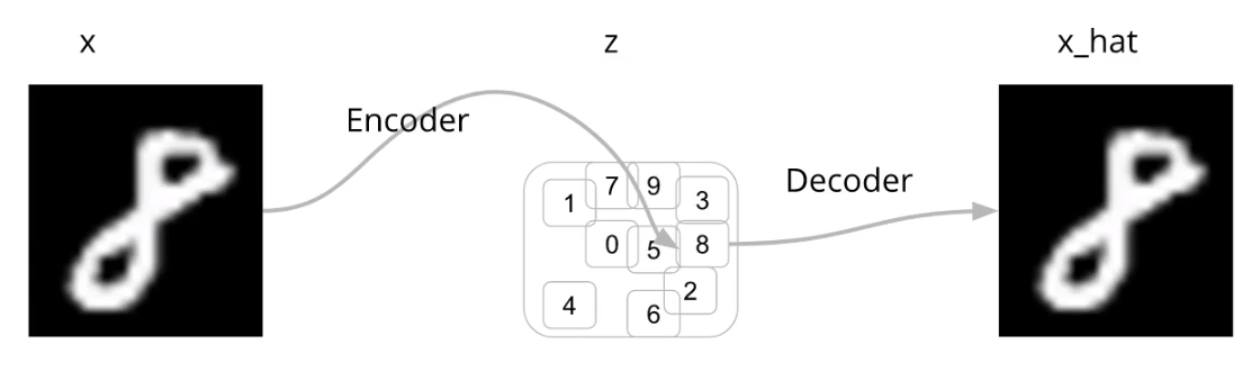### Cost Function of VAE

• Evidence Lower Bound (ELBO) is our objective function that has to be maximized.
• ELBO consists of two terms: Expected Log-Likelihood of the data and KL divergence between q(z|x) and p(z).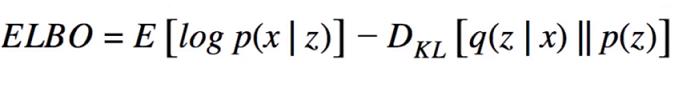• Expected Log-Likelihood is negative cross-entropy between original data and recontructed data.
• "Expected Log-Likelihood encourages the decoder to learn to reconstruct the data. If the decoder’s output does not reconstruct the data well, it will incur a large cost in this loss function".
• If the input and output have Bernoulli distribution, Expected Log-Likelihood can be calculated like this: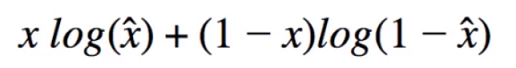• "KL divergence measures how much information is lost (in units of nats) when using q to represent p. It is one measure of how close q is to p".
• KL divergence provides to compare 2 probability distributions.
• If the two probability distributions are exactly same (q=p), KL divergence equals to 0.
• If the two probability distributions are not same (q!=p), KL divergence > 0 .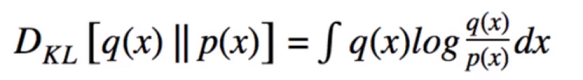• Cost function consists of two part: How the model's output is close to target and regularization.
• COST = (TARGET-OUTPUT) PENALTY-REGULARIZATION PENALTY == RECONSTRUCTION PENALTY - REGULARIZATION PENALTY

• GANs are interesting because it generates samples exceptionally good.
• GANs are used in different applications (details are summarized following sections).
• GANs are different form other generative models (Bayesian Classifier, Variational Autoencoders, Restricted Boltzmann Machines). GANs are not dealing with explicit probabilities, instead, its aim is to reach Nash Equilibrium of a game.
• RBM generate samples with Monte Carlo Sampling (thousands of iterations are needed to generate, and how many iterations are needed is not known). GANs generate samples with in single pass.
• There are 2 different networks in GANs: generator and discriminator, compete against each other.
• Generator Network tries to fool the discriminator.
• Disciminator Network tries not to be fooled.
• Paper: Ian J. Goodfellow, Jean Pouget-Abadie, Mehdi Mirza, Bing Xu, David Warde-Farley, Sherjil Ozair, Aaron Courville, Yoshua Bengio, Generative Adversarial Networks

### GANs Cost Function

#### Discriminator Cost Function

• Generator and Discriminator try to optimize the opposite cost functions.
• Discriminator classifies images as a real or fake images with binary classification.
• t: target; y: output probability of the discriminator.
• Real image: t=1; fake image: t=0; y= p(image is real | image) between (0,1)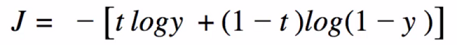• Binary cost function evaluates discriminator cost function.
• x: real images only, x_hat: fake images only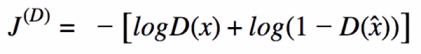• G(z): fake image from generator, x: real image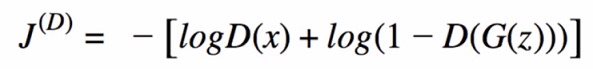• Total cost: summing to individual negative log-likelihoods (batches of data in training)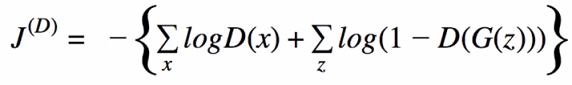• Estimate of the expected value over all possible data
• Discrimator Cost Function: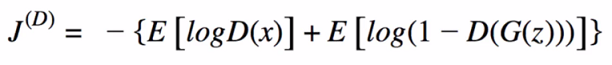#### Generator Cost Function

• Relation between Generator and Discriminator Cost Functions: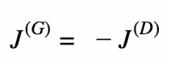• In game theory, this situation is called "zero-sum game". Sum of all players in the game is 0.
• Theta_G: parameters of generator; Theta_D: parameters of discriminator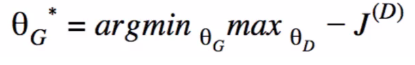• Pseudocode of GANs:
• In the first step: Generator generates some of the real samples and fake samples.
• In the second step: Gradient descent of the discriminator is run one iteration.
• In the third step: Gradient descent of the generator is run one iteration.
• Repeat this until seeing the good samples.
• Some of the researches run third step twice to get better results.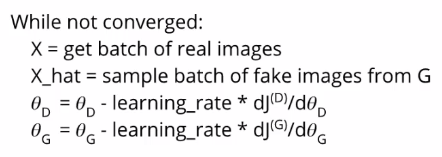### DCGAN

• Paper: Radford, A., Metz, L., and Chintala, S., Unsupervised representation learning with deep convolutional generative adversarial networks
• DCGAN architecture produces high quality and high resolution images in a single pass.
• DCGANs contain batch normalization (batch norm: z=(x-mean)/std, batch norm is used between layers).
• DCGANs also contain only all-convolutional layers instead of contaning convolution, pooling, linear layers together.
• Discriminator uses Leaky-ReLU (Rectified Linear Unit), generator uses normal ReLU.
• Typical Convolution: input size is bigger or equal than output size (Stride>1).
• Deconvolution: input size is smaller than output size (Stride<1).

#### Fractionally-Strided Convolution

• Stride size is smaller than 1.
• If stride=2 is used while convolution operation, output image size is 1/2 original image size.
• If stride=1/2 is used while convolution operation, output image size is 2x original image size.
• With fractionally-strided convolution (deconvolution), output image size is bigger than input image size.

### CycleGAN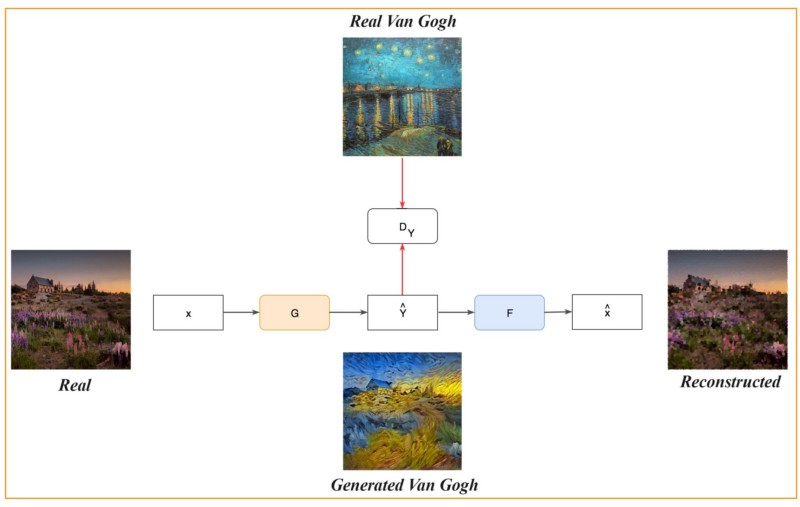### Pix2Pix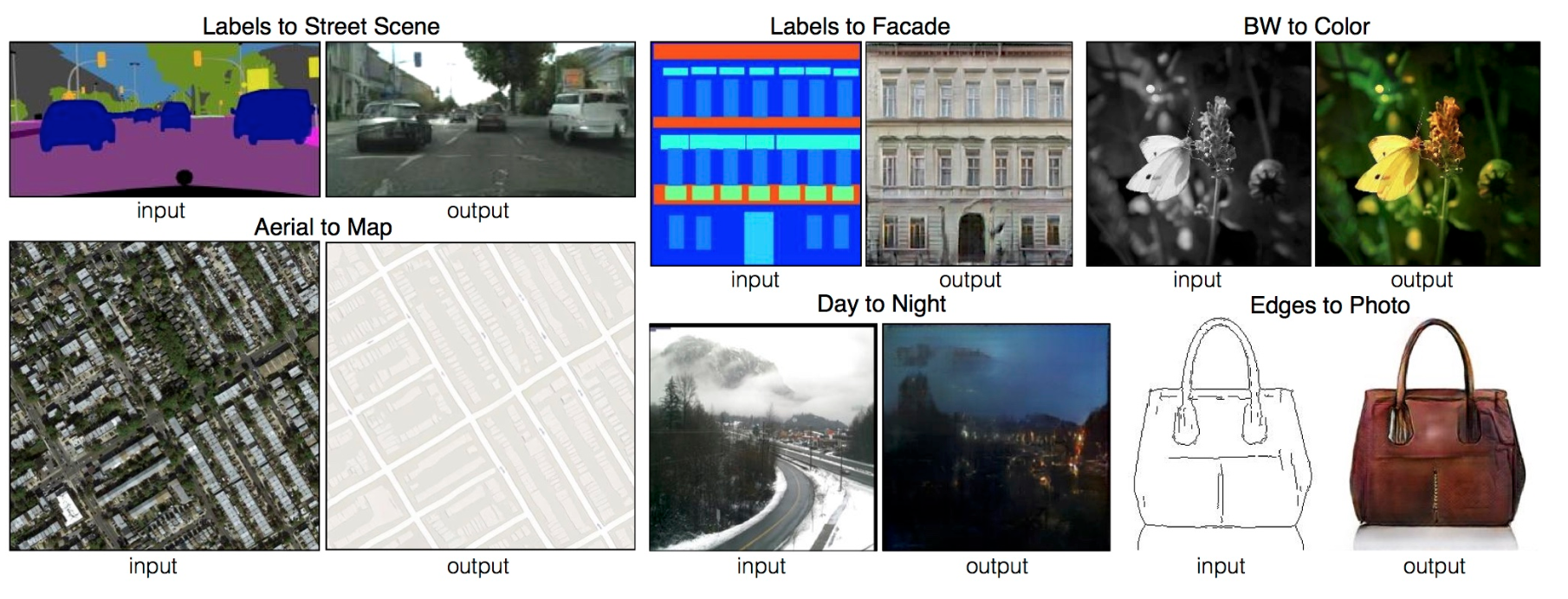### PixelDTGAN

• Pixel-Level Domain Transfer
• PixelDTGAN generates clothing images from an image.
• "The model transfers an input domain to a target domain in semantic level, and generates the target image in pixel level."
• "They verify their model through a challenging task of generating a piece of clothing from an input image of a dressed person"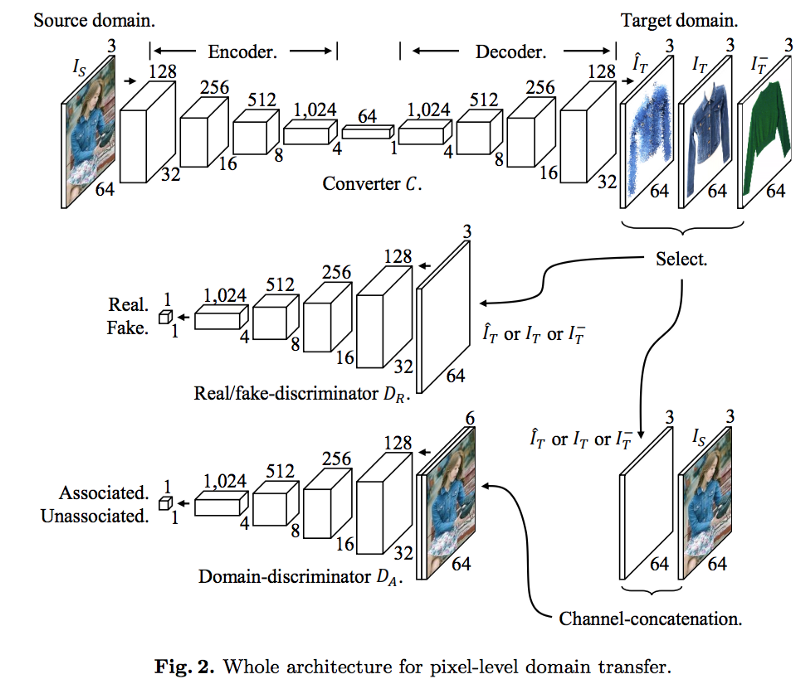### PoseGuided

• Pose Guided Person Image Generation
• "This paper proposes the novel Pose Guided Person Generation Network (PG2 that allows to synthesize person images in arbitrary poses, based on an image of that person and a novel pose"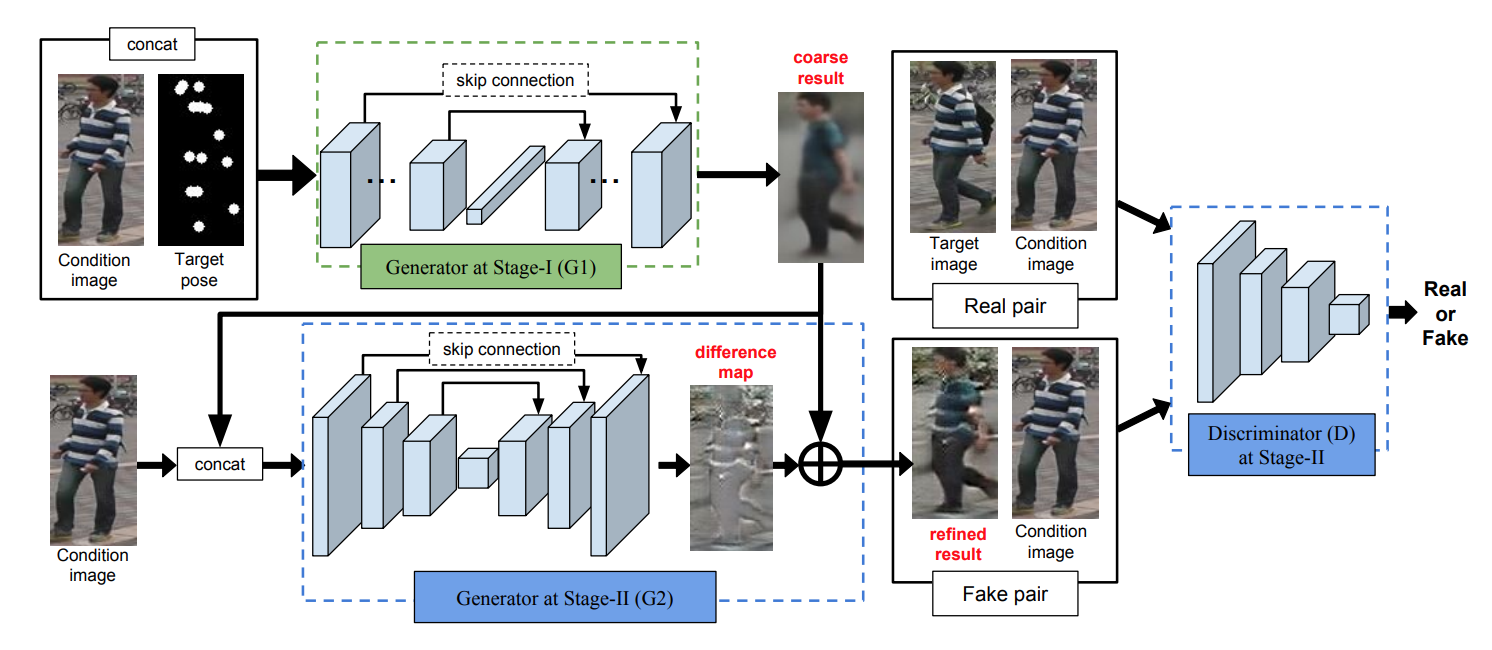### SRGAN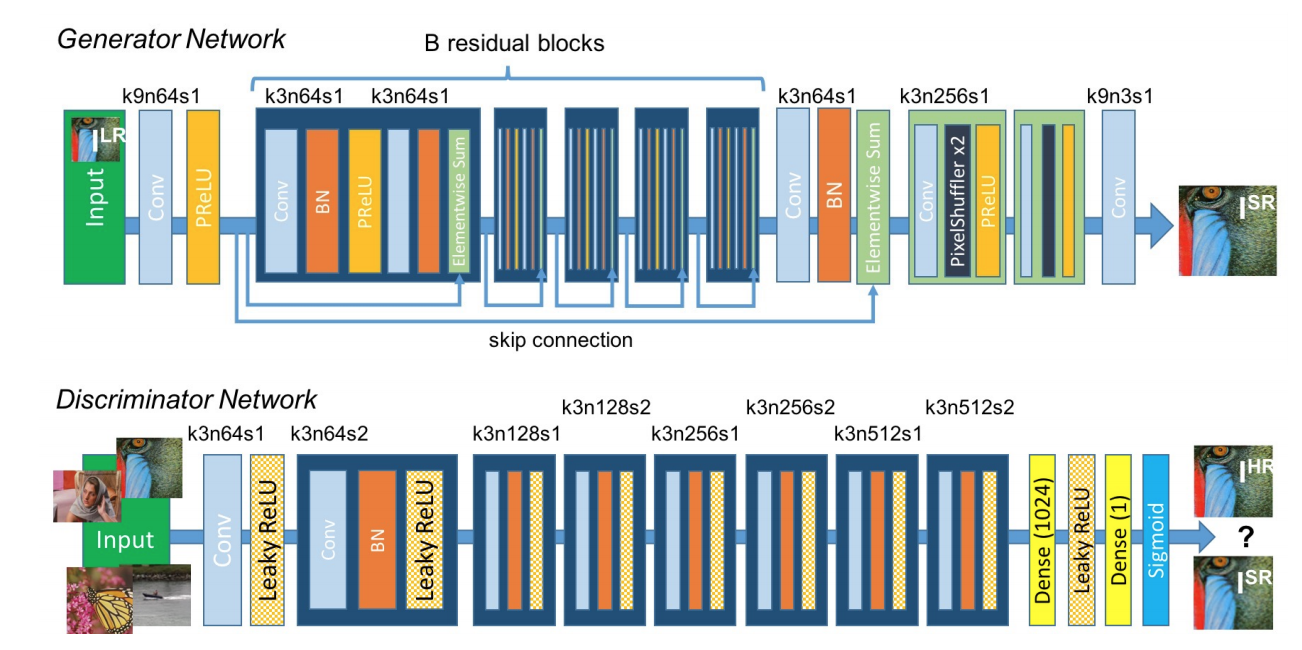### StackGAN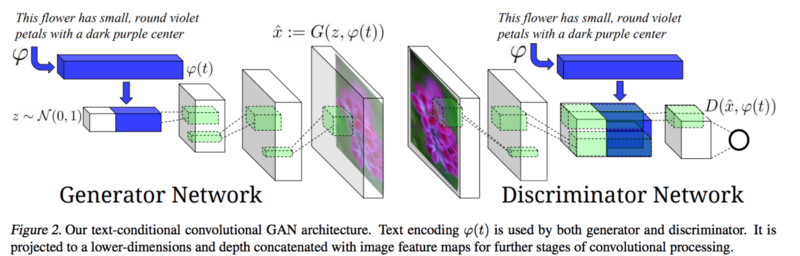### TPGAN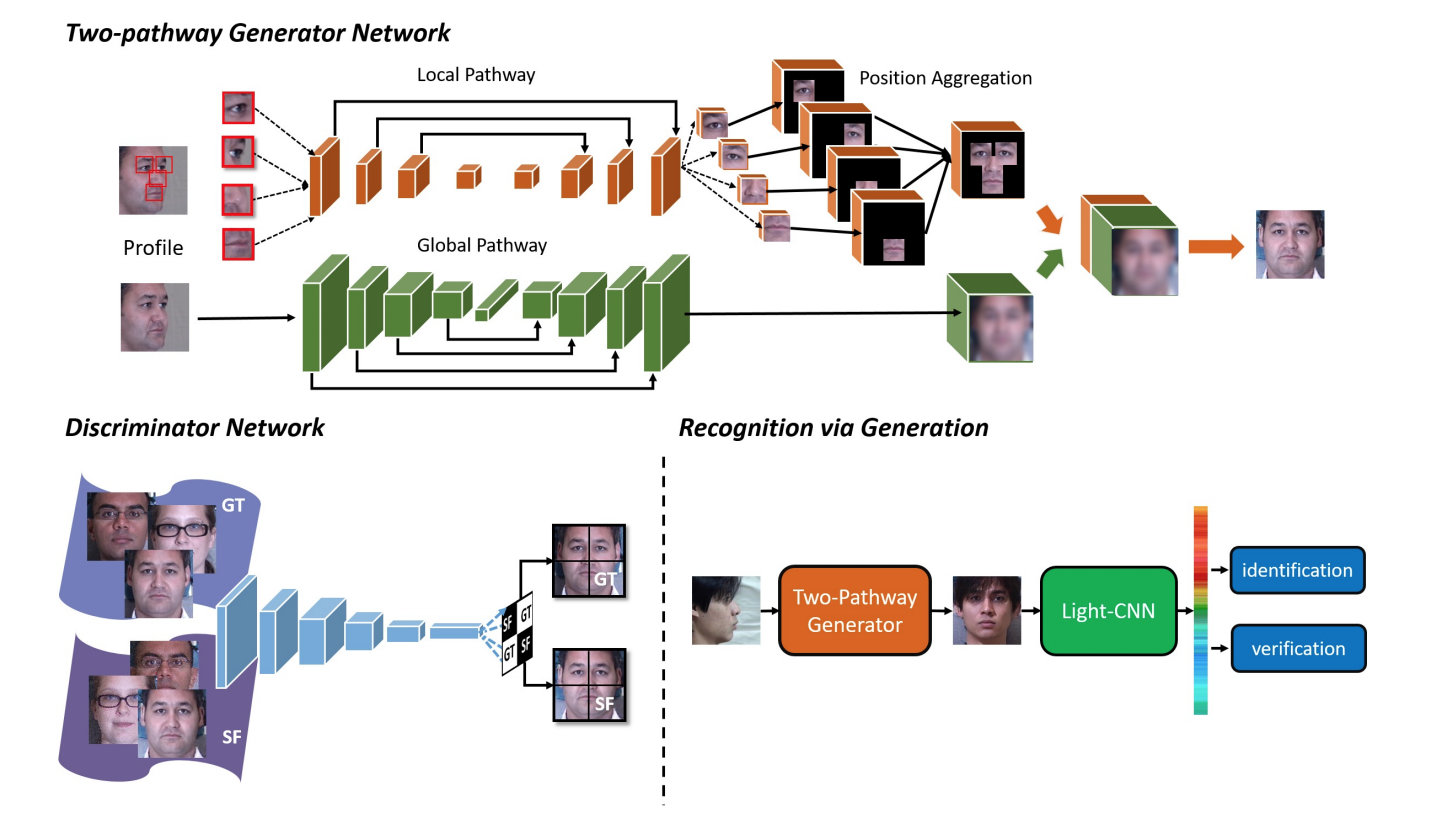### Anime Generation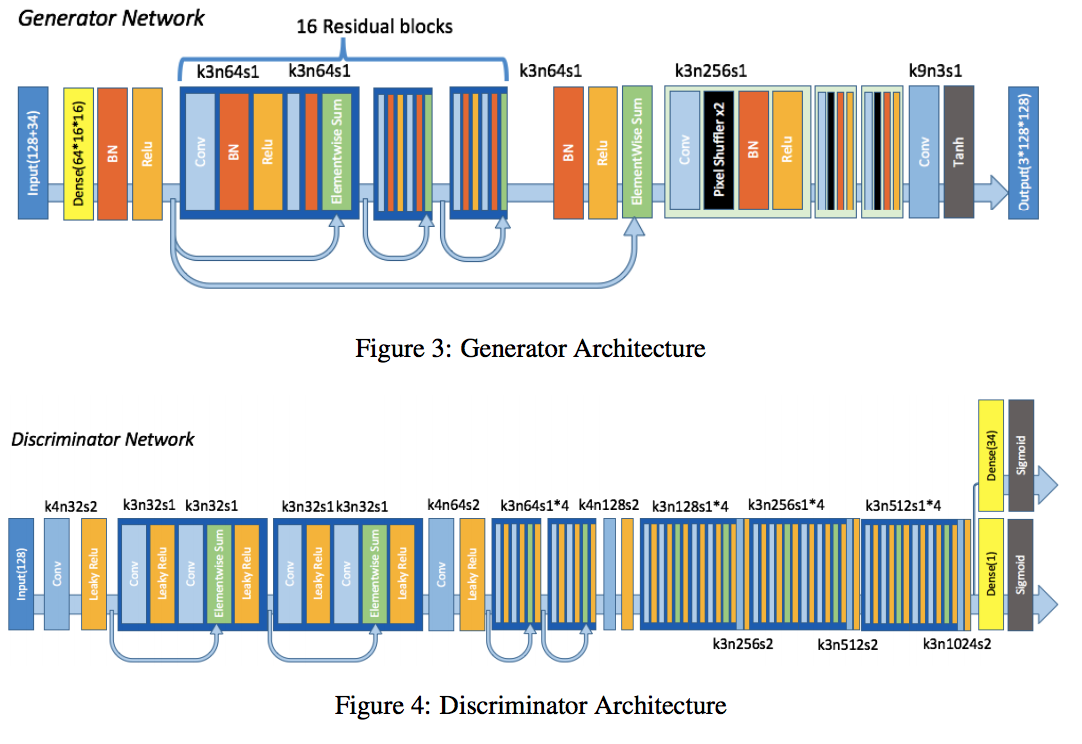### 3DGAN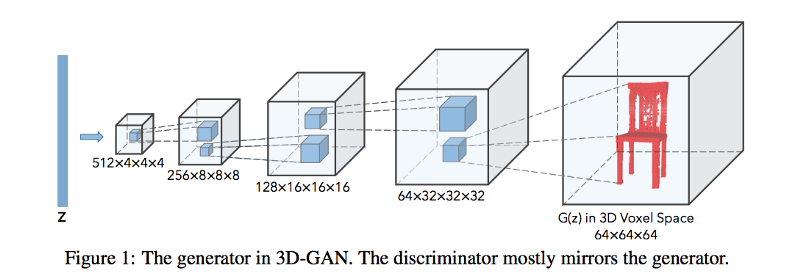### Age-cGAN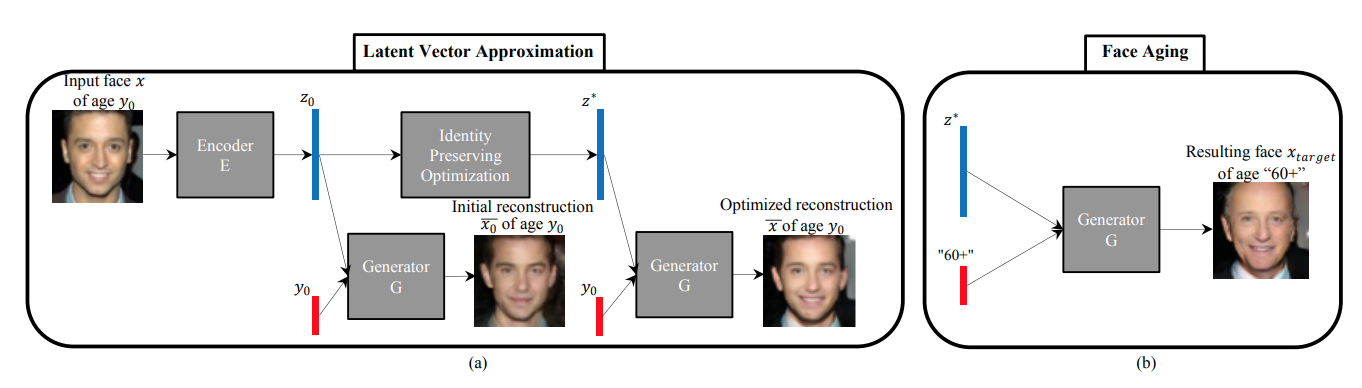### AnoGAN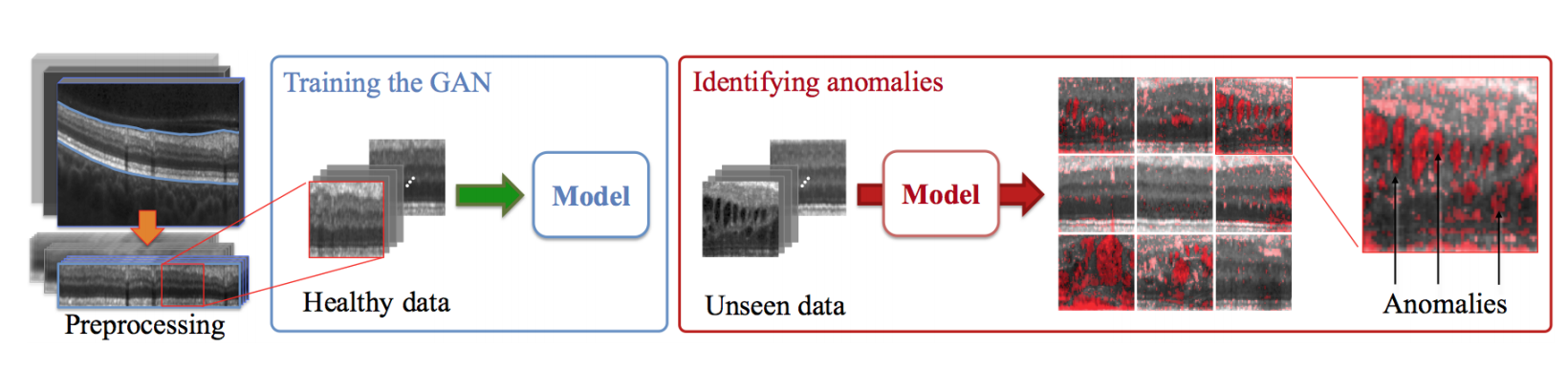### DiscoGAN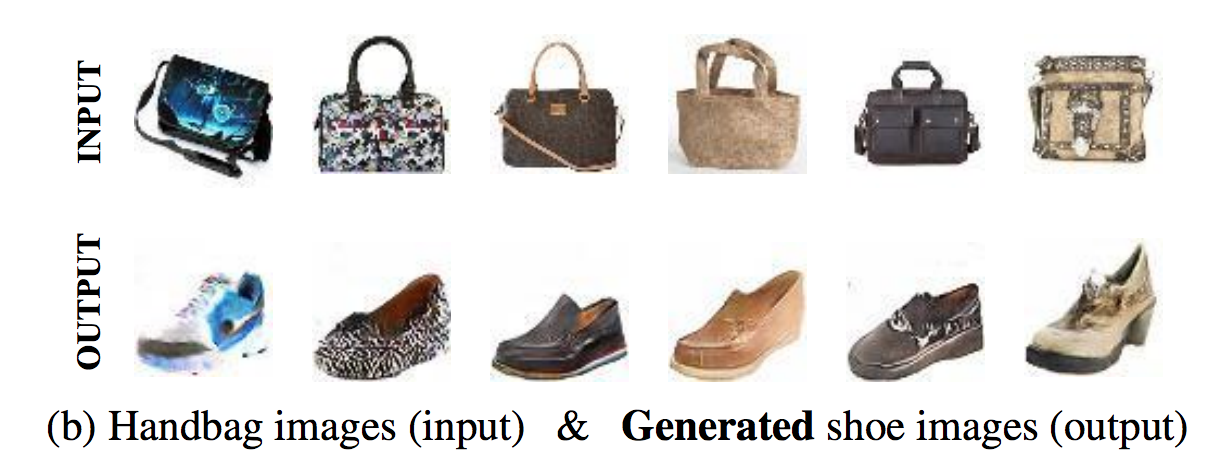### DTN

• Unsupervised Cross-Domain Image Generation
• Proposed method is to create emoji from pictures.
• "They can synthesize an SVHN image that resembles a given MNIST image, or synthesize a face that matches an emoji."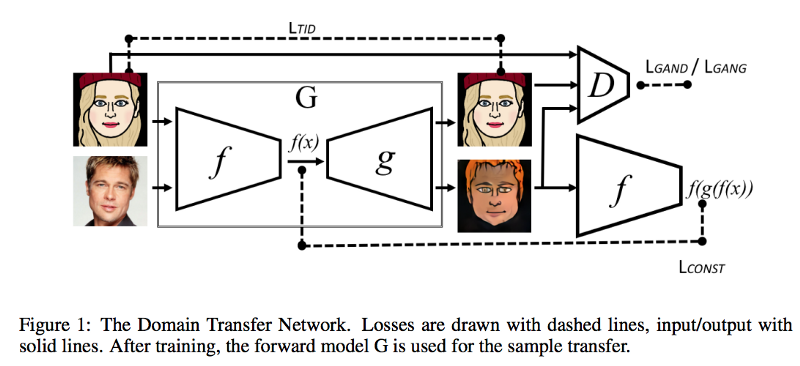### IcGAN

• Invertible Conditional GANs for image editing
• "They evaluate encoders to inverse the mapping of a cGAN, i.e., mapping a real image into a latent space and a conditional representation".
• Proposed method is to reconstruct or edit images with specific attribute.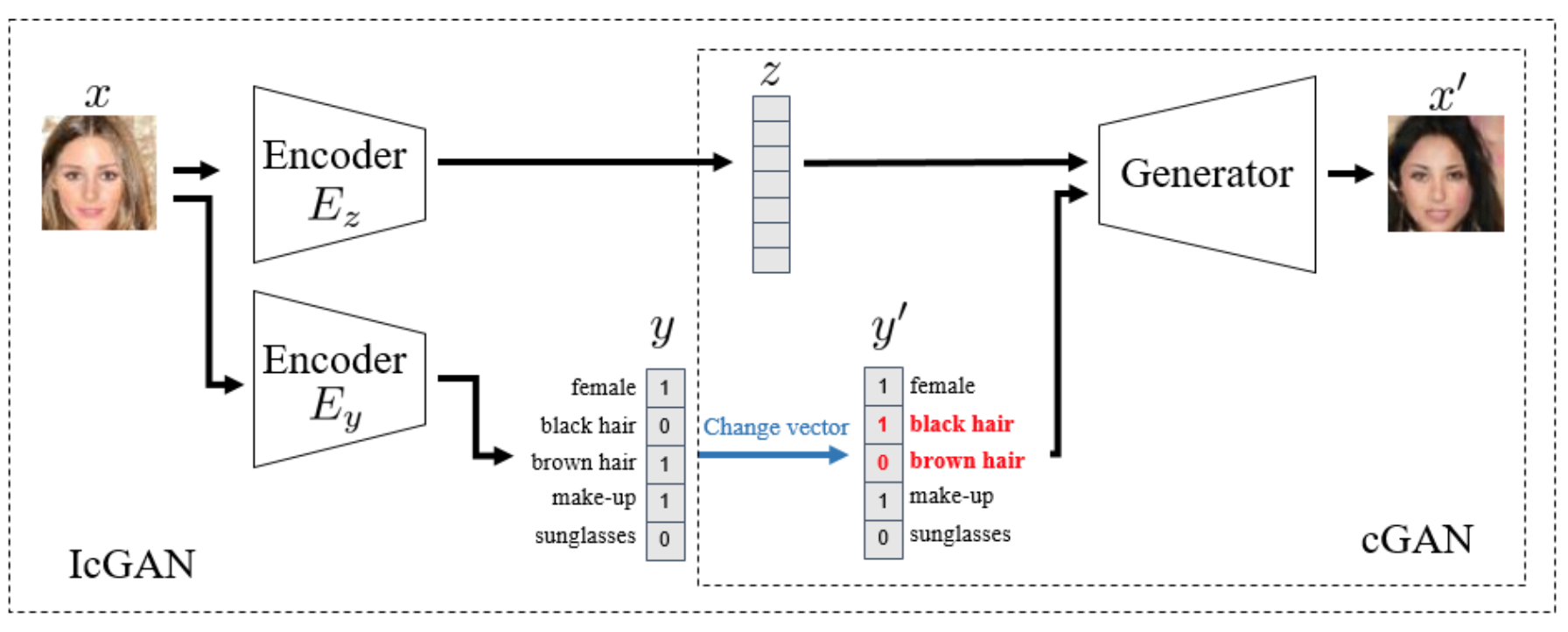### MGAN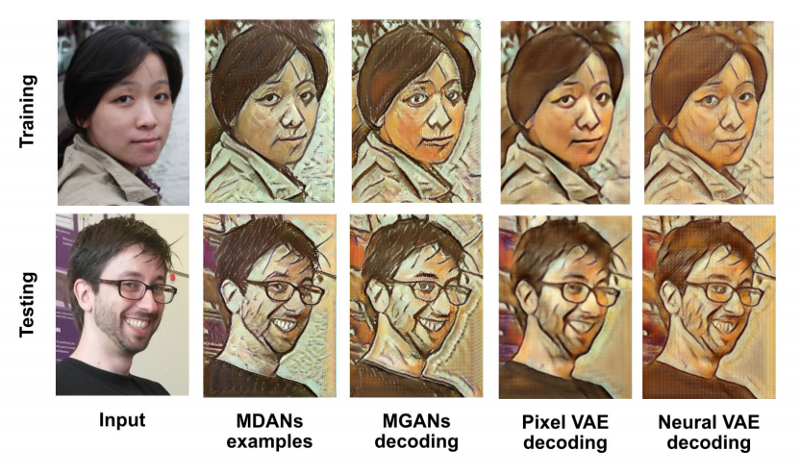### MidiNet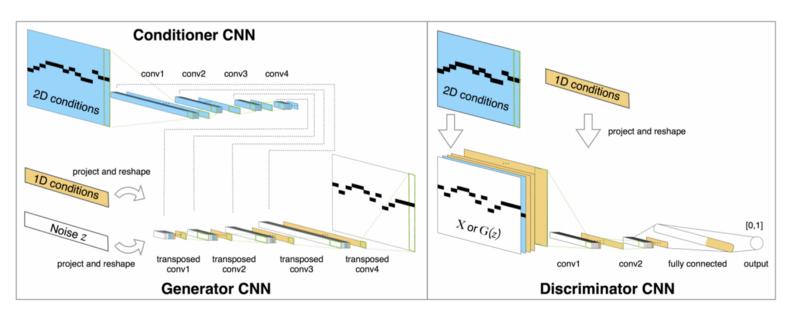### PerceptualGAN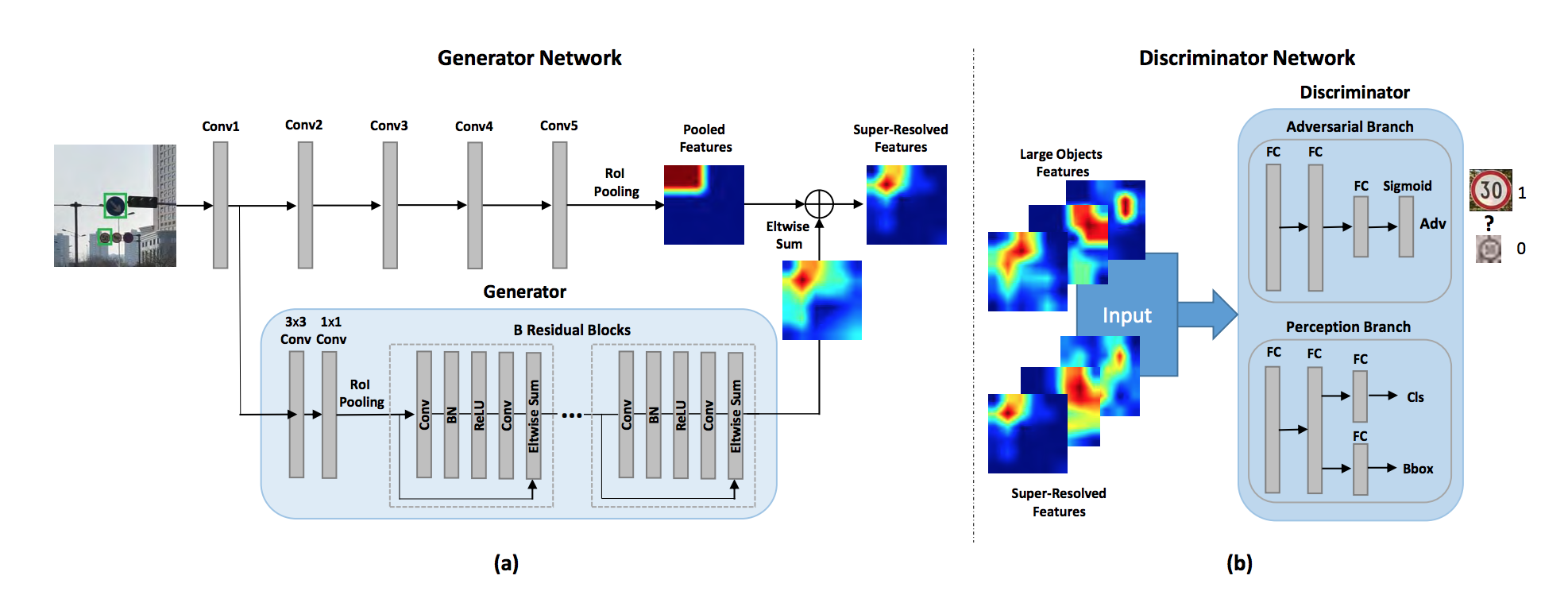## Auto-Regressive Models

• "The basic difference between Generative Adversarial Networks (GANs) and Auto-regressive models is that GANs learn implicit data distribution whereas the latter learns an explicit distribution governed by a prior imposed by model structure" [Sharma].
• Some of the advantages of Auto-Regressive Models over GANs:
• Provides a way to calculate likelihood: They have advantage of returning explicit probability densities. Hence it can be applied in the application areas related compression and probabilistic planning and exploration.
• The training is more stable than GANs:"Training a GAN requires finding the Nash equilibrium". Training of PixelRNN, PixelCNN are more stable than GANs.
• It works for both discrete and continuous data:"It’s hard to learn to generate discrete data for GAN, like text" [Sharma].

### PixelRNN

Paper: Oord et al., Pixel Recurrent Neural Networks (proposed from Google DeepMind)

• It is used for image completion applications.
• "It uses probabilistic density models (like Gaussian or Normal distribution) to quantify the pixels of an image as a product of conditional distributions."
• "This approach turns the modeling problem into a sequence problem wherein the next pixel value is determined by all the previously generated pixel values".
• There are four different methods to implement PixelRNN:
• Row LSTM
• Diagonal BiLSTM
• Fully Convolutional Network
• Multi Scale Network.
• Cost function: "Negative log likelihood (NLL) is used as the loss and evaluation metric as the network predicts(classifies) the values of pixel from values 0–255."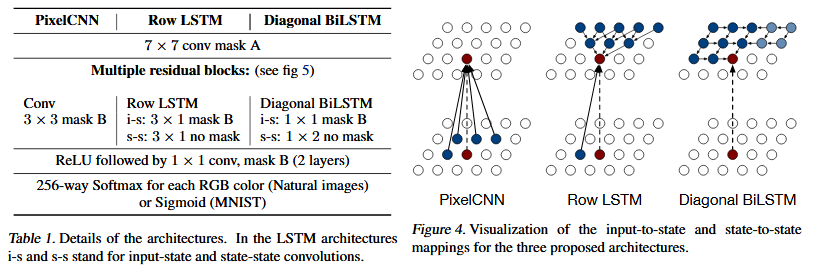### PixelCNN

• Papers: Oord et al., Pixel Recurrent Neural Networks; Oord et al., Conditional Image Generation with PixelCNN Decoders (proposed from Google DeepMind).
• "The main drawback of PixelRNN is that training is very slow as each state needs to be computed sequentially. This can be overcome by using convolutional layers and increasing the receptive field."
• "PixelCNN lowers the training time considerably as compared to PixelRNN."
• "The major drawback of PixelCNN is that it’s performance is worse than PixelRNN. Another drawback is the presence of a Blind Spot in the receptive field"

## Generative Model in Reinforcement Learning

Paper: Jonathan Ho, Stefano Ermon, Generative Adversarial Imitation Learning

• "The standard reinforcement learning setting usually requires one to design a reward function that describes the desired behavior of the agent. However, in practice this can sometimes involve expensive trial-and-error process to get the details right. In contrast, in imitation learning the agent learns from example demonstrations (for example provided by teleoperation in robotics), eliminating the need to design a reward function. This approach can be used to learn policies from expert demonstrations (without rewards) on hard OpenAI Gym environments, such as Ant and Humanoid." [Blog Open-AI].
• "Popular imitation approaches involve a two-stage pipeline: first learning a reward function, then running RL on that reward. Such a pipeline can be slow, and because it’s indirect, it is hard to guarantee that the resulting policy works well. This work shows how one can directly extract policies from data via a connection to GANs" [Blog Open-AI].

## Notes

You can’t perform that action at this time.